Download Presentation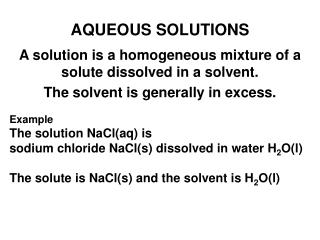AQUEOUS SOLUTIONS

# AQUEOUS SOLUTIONS - PowerPoint PPT Presentation

AQUEOUS SOLUTIONS. Example The solution NaCl(aq) is sodium chloride NaCl(s) dissolved in water H 2 O(l) The solute is NaCl(s) and the solvent is H 2 O(l). A solution is a homogeneous mixture of a solute dissolved in a solvent. The solvent is generally in excess. Types of Solutions.I am the owner, or an agent authorized to act on behalf of the owner, of the copyrighted work described.
Download Presentation## AQUEOUS SOLUTIONS

An Image/Link below is provided (as is) to download presentation

Download Policy: Content on the Website is provided to you AS IS for your information and personal use and may not be sold / licensed / shared on other websites without getting consent from its author.While downloading, if for some reason you are not able to download a presentation, the publisher may have deleted the file from their server.

- - - - - - - - - - - - - - - - - - - - - - - - - - E N D - - - - - - - - - - - - - - - - - - - - - - - - - -
Presentation Transcript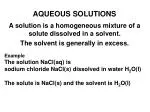### AQUEOUS SOLUTIONS

Example

The solution NaCl(aq) is

sodium chloride NaCl(s) dissolved inwater H2O(l)

The solute is NaCl(s) and the solvent is H2O(l)

A solution is a homogeneous mixture of a solute dissolved in a solvent.

The solvent is generally in excess.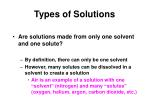Types of Solutions
• Are solutions made from only one solvent and one solute?
• By definition, there can only be one solvent
• However, many solutes can be dissolved in a solvent to create a solution
• Air is an example of a solution with one “solvent” (nitrogen) and many “solutes” (oxygen, helium, argon, carbon dioxide, etc.)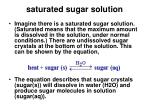saturated sugar solution
• Imagine there is a saturated sugar solution. (Saturated means that the maximum amount is dissolved in the solution, under normal conditions.) There are undissolved sugar crystals at the bottom of the solution. This can be shown by the equation,
• The equation describes that sugar crystals (sugar(s)) will dissolve in water (H2O) and produce sugar molecules in solution (sugar(aq)).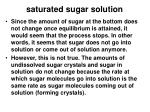saturated sugar solution
• Since the amount of sugar at the bottom does not change once equilibrium is attained, it would seem that the process stops. In other words, it seems that sugar does not go into solution or come out of solution anymore.
• However, this is not true. The amounts of undissolved sugar crystals and sugar in solution do not change because the rate at which sugar molecules go into solution is the same rate as sugar molecules coming out of solution (forming crystals).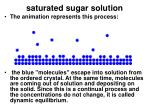saturated sugar solution
• The animation represents this process:
• the blue "molecules" escape into solution from the ordered crystal. At the same time, molecules are coming out of solution and depositing on the solid. Since this is a continual process and the concentrations do not change, it is called dynamic equilibrium.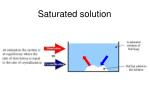### Saturated solution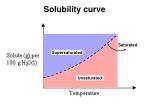Saturated

### Solubility curve

Supersaturated

Unsaturated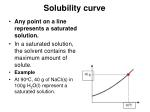Solubility curve
• Any point on a line represents a saturated solution.
• In a saturated solution, the solvent contains the maximum amount of solute.
• Example
• At 90oC, 40 g of NaCl(s) in 100g H2O(l) represent a saturated solution.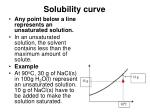Solubility curve
• Any point below a line represents an unsaturated solution.
• In an unsaturated solution, the solvent contains less than the maximum amount of solute.
• Example
• At 90oC, 30 g of NaCl(s) in 100g H2O(l) represent an unsaturated solution. 10 g of NaCl(s) have to be added to make the solution saturated.Solubility curve
• Any point above a line represents a supersaturated solution.
• In a supersaturated solution, the solvent contains more than the maximum amount of solute. A supersaturated solution is very unstable and the amount in excess can precipitate or crystallize.
• Example
• At 90oC, 50 g of NaCl(s) in 100g H2O(l) represent a supersaturated solution. Eventually, 10 g of NaCl(s) will precipitate.### Solubility curve

Any solution can be made saturated, unsaturated, or supersaturated by changing the temperature.### SOLUBILITY

The solubility of a solute in a given amount of solvent is dependent on the

temperature,

the pressure,

and the chemical natures of the solute and solvent.### Temperature

In general, as the temperature of a solution increases the solubility increases.

Increasing the solution temperature allows more sugar to go into solution. Therefore, it is an endothermic process (heat is on the reactant side).Effect of Temperature on Solubility
• The solubility of solutes is dependent on temperature. When a solid dissolves in a liquid, a change in the physical state of the solid analogous to melting takes place. Heat is required to break the bonds holding the molecules in the solid together.
• At the same time, heat is given off during the formation of new solute -- solvent bonds.Increase in solubility with temperature
• If the heat given off in the hydration process is less than the heat required to break apart the solid, the net dissolving reaction is endothermic (energy required). The addition of more heat facilitates the dissolving reaction by providing energy to break bonds in the solid. This is the most common situation where an increase in temperature produces an increase in solubility for solids.
• The use of first-aid instant cold packs is an application of this solubility principle. A salt such as ammonium nitrate is dissolved in water after a sharp blow breaks the containers for each. The dissolving reaction is endothermic - requires heat. Therefore the heat is drawn from the surroundings, the pack feels cold.Molecules in a hot liquid are moving fast. Fast moving molecules have a significant kinetic energy.Decrease in solubility with temperature
• If the heat given off in the hydration process is greater than the heat required to break apart the solid, the net dissolving reaction is exothermic (energy given off).
• The addition of more heat (increases temperature) inhibits the dissolving reaction since excess heat is already being produced by the reaction. This situation is not very common where an increase in temperature produces a decrease in solubility.In a few instances (e.g., Li2SO4 below) the solubility of the salt will decrease with temperature. This observation does not invalidate the above explanantion but rather suggests that several competing ideas need to be taken into account to fully understand chemical processes.Solubility of Gases vs. Temperature
• The variation of solubility for a gas with temperature can be determined by examining the graph below:
• As the temperature increases, the solubility of a gas decrease as shown by the downward trend in the graph .Solubility of Gases vs. Temperature
• More gas is present in a solution with a lower temperature compared to a solution with a higher temperature.
• The reason for this gas solubility relationship with temperature is very similar to the reason that vapor pressure increases with temperature. Increased temperature causes an increase in kinetic energy. The higher kinetic energy causes more motion in molecules which break intermolecular bonds and escape from solution.
• This gas solubility relationship can be remembered if you think about what happens to a "soda pop" as it stands around for awhile at room temperature. The taste is very "flat" since more of the "tangy" carbon dioxide bubbles have escaped. Boiled water also tastes "flat" because all of the oxygen gas has been removed by heating.Summary of temperature effect on solubility
• solid in liquid:
• solubility of an endothermic dissolving solid in a liquid increases with increasing temperature,
• but for an exothermic dissolving one solubility decreases with increasing temperature.
• liquid in liquid:
• for partially dissolving liquids like dimethyl ether(CH3-O-CH3 ) in water (H2O),  solubilty increases with increasing temperature,
• but for a completely dissolving liquids like ethyl alcohol( C2H5OH) in water( H2O) , solubility decreases with increasing temperature.
• gas in liquid:
• solubility of a gas in a liquid almost always decreases with

increasing temperature28g of anhydrous salt per 100g of water.Reading graph: at 84 °C the solubility of potassium sulphate, K2SO4, is

22g per 100g of water.Ex Q1: How much potassium nitrate will dissolve in 20g of water at 34 °C?

• At 34 °C the solubility is 52g per 100g of water, so scaling down, 52 x 20 / 100 = 10.4g will dissolve in 20g of water.Ex Q2: At 25 °C 6.9g of copper sulphate dissolved in 30g of water, what is its solubility in g/100cm3 of water?

• Scaling up, 6.9 x 100 / 30 = 23g/100g of water (check on graph, just less than 23g/100g water).Ex Q3: 200 cm3 of saturated copper sulphate solution was prepared at a temperature of 90 °C. What mass of copper sulphate crystals form if the solution was cooled to 20 °C?

• Solubility of copper sulphate at 90 °C is 67g/100g water, and 21g/100g water at 20 °C. Therefore for mass of crystals formed = 67 - 21 = 46g (for 100 cm3 of solution). However, 200 cm3 of solution was prepared, so total mass of copper sulphate crystallised = 2 x 46 = 92g### Pressure

The solubility of a gas increases as the pressure increases.

Example

Carbon dioxide, CO2(g) in carbonated drinks is dissolved in the solvent by increasing the pressure and also decreasing the temperature.Gas Pressure and Solubility
• Liquids and solids exhibit practically no change of solubility with changes in pressure. Gases as might be expected, increase in solubility with an increase in pressure.
• Henry's Law states that: The solubility of a gas in a liquid is directly proportional to the pressure of that gas above the surface of the solution.
• If the pressure is increased, the gas molecules are "forced" into thesolution since this will best relieve the pressure that has been applied.The number of gas molecules is decreased. The number of gas moleculesdissolved in solution has increased as shown in the graphic on the right.Gas Pressure and Solubility
• Carbonated beverages provide the best example of this phenomena. All carbonated beverages are bottled under pressure to increase the carbon dioxide dissolved in solution.
• When the bottle is opened, the pressure above the solution decreases. As a result, the solution effervesces and some of the carbon dioxide bubbles off.
• Quiz: Champagne continues to ferment in the bottle. The fermentation produces CO2. Why is the cork wired on a bottle of champagne?
• Answer: As more CO2is formed , the pressure of the gas increase.The wire is to prevent the cork from blowing off.Gas Pressure and Solubility
• Deep sea divers may experience a condition called the "bends" if they do not readjust slowly to the lower pressure at the surface.
• As a result of breathing compressed air and being subjected to high pressures caused by water depth, the amount of nitrogen dissolved in blood and other tissues increases.
• If the diver returns to the surface too rapidly, the nitrogen forms bubbles in the blood as it becomes less soluble due to a decrease in pressure. The nitrogen bubbles can cause great pain and possibly death.
• To alleviate this problem somewhat, artificial breathing mixtures of oxygen and helium are used. Helium is only one-fifth as soluble in blood as nitrogen. As a result, there is less dissolved gas to form bubbles.Gas Pressure and Solubility
• Quiz: If a diver had the "bends", describe how this can be treated.
• Answer: Decompression chambers are used to keep a high pressure and gradually lower the pressure.
• Another application of Henry's Law is in the administration of anesthetic gases. If the partial pressure of the anesthetic gas is increased, the anesthetic solubility increases in the blood.Gas Pressure and Solubility
• Quiz: The amount of dissolved oxygen in a mountain lake at10,000 ft and 50oF is __?_ than the amount of dissolved oxygen in a lake near sea level at 50oF.
• Answer: Less at higher altitude because less pressure.
• A Coke at room temperature will have __?_ carbon dioxide in the gas space above the liquid than an ice cold bottle.
• Answer: More gas, because the warm coke can hold less of the gas in solution.Gas Pressure and Solubility
• Hyperbaric therapy, which involves exposure to oxygen at higher than atmospheric pressure may be used to treat hypoxia (low oxygen supply in the tissues). Explain how the treatment works.
• Answer: The increase in pressure in the chamberwill cause more gases to enter into lungs.The rate of solution

The rate of solution is a measure of how fast a substance dissolves. Some of the factors determining the rate of solution are:

• size of the particles -- When a solute dissolves, the action takes place only at the surface of each particle. When the total surface area of the solute particles is increased, the solute dissolves more rapidly. Breaking a solute into smaller pieces increases its surface area and hence its rate of solution. (Sample problem: a cube with sides 1.0 cm long is cut in half, producing two pieces with dimensions of 1.0 cm x 1.0 cm x 0.50 cm. How much greater than the surface area of the original cube is the combined surface areas of the two pieces?
• 2.0 cm2
• stirring -- With liquid and solid solutes, stirring brings fresh portions of the solvent in contact with the solute, thereby increasing the rate of solution.The rate of solution
• amount of solute already dissolved -- When there is little solute already in solution, dissolving takes place relatively rapidly. As the solution approaches the point where no solute can be dissolved, dissolving takes place more slowly.
• temperature -- For solid, liquid and gaseous solutes, changing the temperature not only changes the amount of solute that will dissolve but also changes the rate at which the solute will dissolve.How do I get sugar to dissolve faster in my iced tea?

Stir, and stir, and stir

Fresh solvent contact and interaction with solute

Add sugar to warm tea then add ice

Faster rate of dissolution at higher temperature

Grind the sugar to a powder

Greater surface area, more solute-solvent interaction### Chemical natures of the solute and solvent

A polar solute will dissolve in a polar solvent but not in a nonpolar solvent. The adage "like dissolves like" is very useful.

Example

Alcohol (polar substance) dissolves in water (polar substance)

Water (polar substance) does not dissolve in oil (nonpolar substance)Nature of the solute and solvent

“Likes dissolve likes”

When two similar liquids - here water and methanol- are mixed, the molecules are intermingled.  The mixture has a more disorderly arrangement of molecules than the separate liquids. It is this disordering process that largely drives solution formation.

• polar solute/polar solvent:
• ethanol, salt, sugar in water
• nonpolar solute/nonpolar solvent:
• Iodine in carbontetrachloride, gasoline or benzeneElectrolyte:a substance that conducts electricity when dissolved in water.

Acids, bases and soluble ionic solutions are electrolytes.

Non-electrolyte:a substance that does not conduct electricity when dissolved in water.

Molecular compounds and insoluble ionic compounds are non-electrolytes.

Electrolyte and Non-electrolyteElectrolytes
• Some solutes can dissociate into ions.
• Electric charge can be carried.Types of solutes

high conductivity

Strong Electrolyte -

100% dissociation,

all ions in solution

Na+

Cl-Types of solutes

slight conductivity

Weak Electrolyte -

partial dissociation,

molecules and ions in solution

CH3COOH

CH3COO-

H+Types of solutes

no conductivity

Non-electrolyte -

No dissociation,

all molecules in solution

sugarTypes of Electrolytes
• Strong electrolyte dissociates completely.
• Good electrical conduction.
• Weak electrolyte partially dissociates.
• Fair conductor of electricity.
• Non-electrolyte does not dissociate.
• Poor conductor of electricity.A weak electrolyte:

CH3COOH(aq)← CH3COO-(aq) +H+(aq)

A non-electrolyte:

CH3OH(aq)

Representation of Electrolytes using Chemical Equations

MgCl2(s) → Mg2+(aq) + 2 Cl-(aq)

A strong electrolyte:Strong Electrolytes

Strong acids: HNO3, H2SO4, HCl, HClO4

Strong bases: MOH (M = Na, K, Cs, Rb etc)

Salts: All salts dissolving in water are completely ionized.

Stoichiometry & concentration relationship

NaCl (s)  Na+ (aq) + Cl– (aq)

Ca(OH)2 (s) Ca2+(aq) + 2 OH– (aq)

AlCl3 (s)  Al3+ (aq) + 3 Cl– (aq)

(NH4)2SO4 (s)  2 NH4+ (aq) + SO42– (aq)Acid-base Reactions

HCl (g)  H+ (aq) + Cl– (aq)

NaOH (s)  Na+ (aq) + OH– (aq)

neutralization reaction: H+ (aq) + OH– (aq) H2O (l)

Explain these reactions

Mg(OH)2 (s) + 2 H+ Mg2+ (aq) + 2 H2O (l)

CaCO3 (s) + 2 H+ Ca2+ (aq) + H2O (l) + CO2 (g)

Mg(OH)2 (s) + 2 HC2H3O2  Mg2+ (aq) + 2 H2O (l) + 2 C2H3O2 – (aq) acetic acidPrecipitation Reactions

Heterogeneous Reactions

Spectator ions or bystander ions

Ag+ (aq) + NO3– (aq) + Cs+ (aq) + I– (aq)  AgI (s) + NO3– (aq) + Cs+ (aq)

Ag+ (aq) + I– (aq)  AgI (s) (net reaction)or Ag+ + I– AgI (s)

Mostly insoluble

Silver halidesMetal sulfides, hydroxidescarbonates, phosphates

Soluble ions

Alkali metals, NH4+nitrates, ClO4-, acetate

Mostly soluble ions

Halides, sulfatesSpectator ions

Ag+(aq) + NO3-(aq) + Na+(aq) + I-(aq) →

AgI(s) + Na+(aq) + NO3-(aq)

Net Ionic Equation

Overall Precipitation Reaction:

AgNO3(aq) +NaI (aq) → AgI(s) + NaNO3(aq)

Complete ionic equation:

Ag+(aq) + NO3-(aq) + Na+(aq) + I-(aq) →

AgI(s) + Na+(aq) + NO3-(aq)

Net ionic equation:

Ag+(aq) + I-(aq) → AgI(s)Suppose copper (II) sulfate reacts with sodium sulfide.

Write out the chemical reaction and name the precipitate.

CuSO4 (aq) + Na2S (aq) CuS (s) + Na2SO4 (aq)

Write out the net ionic equation.

Cu+2(aq)SO4-2 (aq) + 2Na+ (aq) + S-2(aq) CuS (s) + 2Na+ + SO4-2 (aq)

Cu+2(aq)+ S-2(aq) CuS (s)

Suppose potassium hydroxide reacts with magnesium chloride.

Write out the reaction and name the precipitate.

Write out the net ionic equation.

How to write chemical equationsg solute

g solute

x 100

x 100

=

g solution

g solute + g solvent

moles of solute

volume in liters of solution

Units of Concentrations

amount of solute per amount of solvent or solution

Percent (by mass) =

Molarity (M) =

moles = M x VLExamples

What is the percent of KCl if 15 g KCl are

placed in 75 g water?

%KCl = 15g x 100/(15 g + 75 g) = 17%

What is the molarity of the KCl if 90 mL of

solution are formed?

mole KCl = 15 g x (1 mole/74.5 g) = 0.20 mole

molarity = 0.20 mole/0.090L = 2.2 M KClExamples:

Example 1:What is the concentration when 5.2 moles of hydrosulfuric acid are dissolved in 500 mL of water?

Step one: Convert volume to liters, mass to moles.

500 mL = 0.500 L

Step two: Calculate concentration.

C = 5.2 mol/0.500 L

= 10mol/LExample 2:

What is the volume when 9.0 moles are present in 5.6 mol/L hydrochloric acid?

• Example 3:

How many moles are present in 450 mL of 1.5 mol/L calcium hydroxide?

• Example 4:

What is the concentration of 5.6 g of magnesium hydroxide dissolved in 550 mL?

• Example 5:

What is the volume of a 0.100 mol/L solution that contains 5.0 g of sodium chloride?How many Tums tablets, each 500 mg CaCO3, would it take to neutralize a quart of vinegar, 0.83 M acetic acid (CH3COOH)?

2CH3COOH(aq) + CaCO3(s)  Ca(CH3COO)2(aq) + H2O + CO2(g)

a quart

moles acetic acid = 0.83 moles/L x 0.95 L = 0.79 moles AA

the mole ratio

mole CaCO3 = 0.79 moles AA x (1 mole CaCO3/2 moles AA)

= 0.39 moles CaCO3

molar mass

mass CaCO3 = 0.39 moles x 100 g/mole = 39 g CaCO3

number of tablets = 39 g x (1 tablet/0.500g) = 79 tablets